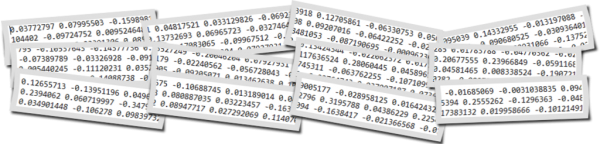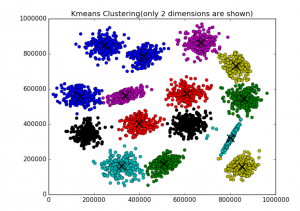## Text Clustering with Word Embedding in Machine LearningText clustering is widely used in many applications such as recommender systems, sentiment analysis, topic selection, user segmentation. Word embeddings (for example word2vec) allow to exploit ordering
of the words and semantics information from the text corpus. In this blog you can find several posts dedicated different word embedding models:

In contrast to last post from the above list, in this post we will discover how to do text clustering with word embeddings at sentence (phrase) level. The sentence could be a few words, phrase or paragraph like tweet. For examples we have 1000 of tweets and want to group in several clusters. So each cluster would contain one or more tweets.

## Data

Our data will be the set of sentences (phrases) containing 2 topics as below:
Note: I highlighted in bold 3 sentences on weather topic, all other sentences have totally different topic.
sentences = [[‘this’, ‘is’, ‘the’, ‘one’,’good’, ‘machine’, ‘learning’, ‘book’],
[‘this’, ‘is’, ‘another’, ‘book’],
[‘one’, ‘more’, ‘book’],
[‘weather’, ‘rain’, ‘snow’],
[‘yesterday’, ‘weather’, ‘snow’],
[‘forecast’, ‘tomorrow’, ‘rain’, ‘snow’],

[‘this’, ‘is’, ‘the’, ‘new’, ‘post’],
[‘this’, ‘is’, ‘about’, ‘more’, ‘machine’, ‘learning’, ‘post’],
[‘and’, ‘this’, ‘is’, ‘the’, ‘one’, ‘last’, ‘post’, ‘book’]]

## Word Embedding Method

For embeddings we will use gensim word2vec model. There is also doc2vec model – but we will use it at next post.
With the need to do text clustering at sentence level there will be one extra step for moving from word level to sentence level. For each sentence from the set of sentences, word embedding of each word is summed and in the end divided by number of words in the sentence. So we are getting average of all word embeddings for each sentence and use them as we would use embeddings at word level – feeding to machine learning clustering algorithm such k-means.

Here is the example of the function that doing this:

```def sent_vectorizer(sent, model):
sent_vec =[]
numw = 0
for w in sent:
try:
if numw == 0:
sent_vec = model[w]
else:
numw+=1
except:
pass

return np.asarray(sent_vec) / numw
```

Now we will use text clustering Kmeans algorithm with word2vec model for embeddings. For kmeans algorithm we will use 2 separate implementations with different libraries NLTK for KMeansClusterer and sklearn for cluster. This was described in previous posts (see the list above).

The code for this article can be found in the end of this post. We use 2 for number of clusters in both k means text clustering algorithms.
Additionally we will plot data using tSNE.

## Output

Below are results

[1, 1, 1, 0, 0, 0, 1, 1, 1]

Cluster id and sentence:
1:[‘this’, ‘is’, ‘the’, ‘one’, ‘good’, ‘machine’, ‘learning’, ‘book’]
1:[‘this’, ‘is’, ‘another’, ‘book’]
1:[‘one’, ‘more’, ‘book’]
0:[‘weather’, ‘rain’, ‘snow’]
0:[‘yesterday’, ‘weather’, ‘snow’]
0:[‘forecast’, ‘tomorrow’, ‘rain’, ‘snow’]

1:[‘this’, ‘is’, ‘the’, ‘new’, ‘post’]
1:[‘this’, ‘is’, ‘about’, ‘more’, ‘machine’, ‘learning’, ‘post’]
1:[‘and’, ‘this’, ‘is’, ‘the’, ‘one’, ‘last’, ‘post’, ‘book’]

Score (Opposite of the value of X on the K-means objective which is Sum of distances of samples to their closest cluster center):
-0.0008175040203510163
Silhouette_score:
0.3498247

Cluster id and sentence:
1 [‘this’, ‘is’, ‘the’, ‘one’, ‘good’, ‘machine’, ‘learning’, ‘book’]
1 [‘this’, ‘is’, ‘another’, ‘book’]
1 [‘one’, ‘more’, ‘book’]
0 [‘weather’, ‘rain’, ‘snow’]
0 [‘yesterday’, ‘weather’, ‘snow’]
0 [‘forecast’, ‘tomorrow’, ‘rain’, ‘snow’]

1 [‘this’, ‘is’, ‘the’, ‘new’, ‘post’]
1 [‘this’, ‘is’, ‘about’, ‘more’, ‘machine’, ‘learning’, ‘post’]
1 [‘and’, ‘this’, ‘is’, ‘the’, ‘one’, ‘last’, ‘post’, ‘book’]

We see that the data were clustered according to our expectation – different sentences by topic appeared to different clusters. Thus we learned how to do clustering algorithms in data mining or machine learning with word embeddings at sentence level. Here we used kmeans clustering and word2vec embedding model. We created additional function to go from word embeddings to sentence embeddings level. In the next post we will use doc2vec and will not need this function.

Below is full source code python script.

```from gensim.models import Word2Vec

from nltk.cluster import KMeansClusterer
import nltk
import numpy as np

from sklearn import cluster
from sklearn import metrics

# training data

sentences = [['this', 'is', 'the', 'one','good', 'machine', 'learning', 'book'],
['this', 'is',  'another', 'book'],
['one', 'more', 'book'],
['weather', 'rain', 'snow'],
['yesterday', 'weather', 'snow'],
['forecast', 'tomorrow', 'rain', 'snow'],
['this', 'is', 'the', 'new', 'post'],
['this', 'is', 'about', 'more', 'machine', 'learning', 'post'],
['and', 'this', 'is', 'the', 'one', 'last', 'post', 'book']]

model = Word2Vec(sentences, min_count=1)

def sent_vectorizer(sent, model):
sent_vec =[]
numw = 0
for w in sent:
try:
if numw == 0:
sent_vec = model[w]
else:
numw+=1
except:
pass

return np.asarray(sent_vec) / numw

X=[]
for sentence in sentences:
X.append(sent_vectorizer(sentence, model))

print ("========================")
print (X)

# note with some version you would need use this (without wv)
#  model[model.vocab]
print (model[model.wv.vocab])

print (model.similarity('post', 'book'))
print (model.most_similar(positive=['machine'], negative=[], topn=2))

NUM_CLUSTERS=2
kclusterer = KMeansClusterer(NUM_CLUSTERS, distance=nltk.cluster.util.cosine_distance, repeats=25)
assigned_clusters = kclusterer.cluster(X, assign_clusters=True)
print (assigned_clusters)

for index, sentence in enumerate(sentences):
print (str(assigned_clusters[index]) + ":" + str(sentence))

kmeans = cluster.KMeans(n_clusters=NUM_CLUSTERS)
kmeans.fit(X)

labels = kmeans.labels_
centroids = kmeans.cluster_centers_

print ("Cluster id labels for inputted data")
print (labels)
print ("Centroids data")
print (centroids)

print ("Score (Opposite of the value of X on the K-means objective which is Sum of distances of samples to their closest cluster center):")
print (kmeans.score(X))

silhouette_score = metrics.silhouette_score(X, labels, metric='euclidean')

print ("Silhouette_score: ")
print (silhouette_score)

import matplotlib.pyplot as plt

from sklearn.manifold import TSNE

model = TSNE(n_components=2, random_state=0)
np.set_printoptions(suppress=True)

Y=model.fit_transform(X)

plt.scatter(Y[:, 0], Y[:, 1], c=assigned_clusters, s=290,alpha=.5)

for j in range(len(sentences)):
plt.annotate(assigned_clusters[j],xy=(Y[j], Y[j]),xytext=(0,0),textcoords='offset points')
print ("%s %s" % (assigned_clusters[j],  sentences[j]))

plt.show()
```

## K Means Clustering Example with Word2Vec in Data Mining or Machine Learning

In this post you will find K means clustering example with word2vec in python code. Word2Vec is one of the popular methods in language modeling and feature learning techniques in natural language processing (NLP). This method is used to create word embeddings in machine learning whenever we need vector representation of data.

For example in data clustering algorithms instead of bag of words (BOW) model we can use Word2Vec. The advantage of using Word2Vec is that it can capture the distance between individual words.

The example in this post will demonstrate how to use results of Word2Vec word embeddings in clustering algorithms. For this, Word2Vec model will be feeded into several K means clustering algorithms from NLTK and Scikit-learn libraries.Here we will do clustering at word level. Our clusters will be groups of words. In case we need to cluster at sentence or paragraph level, here is the link that showing how to move from word level to sentence/paragraph level:

Text Clustering with Word Embedding in Machine Learning

There is also doc2vec word embedding model that is based on word2vec. doc2vec is created for embedding sentence/paragraph/document. Here is the link how to use doc2vec word embedding in machine learning:
Text Clustering with doc2vec Word Embedding Machine Learning Model

## Getting Word2vec

Using word2vec from python library gensim is simple and well described in tutorials and on the web , , . Here we just look at basic example. For the input we use the sequence of sentences hard-coded in the script.

```from gensim.models import Word2Vec
sentences = [['this', 'is', 'the', 'good', 'machine', 'learning', 'book'],
['this', 'is',  'another', 'book'],
['one', 'more', 'book'],
['this', 'is', 'the', 'new', 'post'],
['this', 'is', 'about', 'machine', 'learning', 'post'],
['and', 'this', 'is', 'the', 'last', 'post']
model = Word2Vec(sentences, min_count=1)
```

Now we have model with words embedded. We can query model for similar words like below or ask to represent word as vector:

```print (model.similarity('this', 'is'))
print (model.similarity('post', 'book'))
#output -0.0198180344218
#output -0.079446731287
print (model.most_similar(positive=['machine'], negative=[], topn=2))
#output: [('new', 0.24608060717582703), ('is', 0.06899910420179367)]
print (model['the'])
#output [-0.00217354 -0.00237131  0.00296396 ...,  0.00138597  0.00291924  0.00409528]
```

To get vocabulary or the number of words in vocabulary:

```print (list(model.vocab))
print (len(list(model.vocab)))
```

This will produce: [‘good’, ‘this’, ‘post’, ‘another’, ‘learning’, ‘last’, ‘the’, ‘and’, ‘more’, ‘new’, ‘is’, ‘one’, ‘about’, ‘machine’, ‘book’]

Now we will feed word embeddings into clustering algorithm such as k Means which is one of the most popular unsupervised learning algorithms for finding interesting segments in the data. It can be used for separating customers into groups, combining documents into topics and for many other applications.

You will find below two k means clustering examples.

K Means Clustering with NLTK Library
Our first example is using k means algorithm from NLTK library.
To use word embeddings word2vec in machine learning clustering algorithms we initiate X as below:

```X = model[model.vocab]
```

Now we can plug our X data into clustering algorithms.

```from nltk.cluster import KMeansClusterer
import nltk
NUM_CLUSTERS=3
kclusterer = KMeansClusterer(NUM_CLUSTERS, distance=nltk.cluster.util.cosine_distance, repeats=25)
assigned_clusters = kclusterer.cluster(X, assign_clusters=True)
print (assigned_clusters)
# output: [0, 2, 1, 2, 2, 1, 2, 2, 0, 1, 0, 1, 2, 1, 2]
```

In the python code above there are several options for the distance as below:

nltk.cluster.util.cosine_distance(u, v)
Returns 1 minus the cosine of the angle between vectors v and u. This is equal to 1 – (u.v / |u||v|).

nltk.cluster.util.euclidean_distance(u, v)
Returns the euclidean distance between vectors u and v. This is equivalent to the length of the vector (u – v).

Here we use cosine distance to cluster our data.
After we got cluster results we can associate each word with the cluster that it got assigned to:

```words = list(model.vocab)
for i, word in enumerate(words):
print (word + ":" + str(assigned_clusters[i]))
```

Here is the output for the above:
good:0
this:2
post:1
another:2
learning:2
last:1
the:2
and:2
more:0
new:1
is:0
one:1
machine:1
book:2

## K Means Clustering with Scikit-learn Library

This example is based on k means from scikit-learn library.

```from sklearn import cluster
from sklearn import metrics
kmeans = cluster.KMeans(n_clusters=NUM_CLUSTERS)
kmeans.fit(X)

labels = kmeans.labels_
centroids = kmeans.cluster_centers_

print ("Cluster id labels for inputted data")
print (labels)
print ("Centroids data")
print (centroids)

print ("Score (Opposite of the value of X on the K-means objective which is Sum of distances of samples to their closest cluster center):")
print (kmeans.score(X))

silhouette_score = metrics.silhouette_score(X, labels, metric='euclidean')

print ("Silhouette_score: ")
print (silhouette_score)
```

In this example we also got some useful metrics to estimate clustering performance.
Output:

```Cluster id labels for inputted data
[0 1 1 ..., 1 2 2]
Centroids data
[[ -3.82586889e-04   1.39791325e-03  -2.13839358e-03 ...,  -8.68172920e-04
-1.23599875e-03   1.80053393e-03]
[ -3.11774168e-04  -1.63297475e-03   1.76715955e-03 ...,  -1.43826099e-03
1.22940990e-03   1.06353679e-03]
[  1.91571176e-04   6.40696089e-04   1.38173658e-03 ...,  -3.26442620e-03
-1.08828480e-03  -9.43636987e-05]]

Score (Opposite of the value of X on the K-means objective which is Sum of distances of samples to their closest cluster center):
-0.00894730946094
Silhouette_score:
0.0427737
```

Here is the full python code of the script.

```# -*- coding: utf-8 -*-

from gensim.models import Word2Vec

from nltk.cluster import KMeansClusterer
import nltk

from sklearn import cluster
from sklearn import metrics

# training data

sentences = [['this', 'is', 'the', 'good', 'machine', 'learning', 'book'],
['this', 'is',  'another', 'book'],
['one', 'more', 'book'],
['this', 'is', 'the', 'new', 'post'],
['this', 'is', 'about', 'machine', 'learning', 'post'],
['and', 'this', 'is', 'the', 'last', 'post']]

# training model
model = Word2Vec(sentences, min_count=1)

# get vector data
X = model[model.vocab]
print (X)

print (model.similarity('this', 'is'))

print (model.similarity('post', 'book'))

print (model.most_similar(positive=['machine'], negative=[], topn=2))

print (model['the'])

print (list(model.vocab))

print (len(list(model.vocab)))

NUM_CLUSTERS=3
kclusterer = KMeansClusterer(NUM_CLUSTERS, distance=nltk.cluster.util.cosine_distance, repeats=25)
assigned_clusters = kclusterer.cluster(X, assign_clusters=True)
print (assigned_clusters)

words = list(model.vocab)
for i, word in enumerate(words):
print (word + ":" + str(assigned_clusters[i]))

kmeans = cluster.KMeans(n_clusters=NUM_CLUSTERS)
kmeans.fit(X)

labels = kmeans.labels_
centroids = kmeans.cluster_centers_

print ("Cluster id labels for inputted data")
print (labels)
print ("Centroids data")
print (centroids)

print ("Score (Opposite of the value of X on the K-means objective which is Sum of distances of samples to their closest cluster center):")
print (kmeans.score(X))

silhouette_score = metrics.silhouette_score(X, labels, metric='euclidean')

print ("Silhouette_score: ")
print (silhouette_score)
```